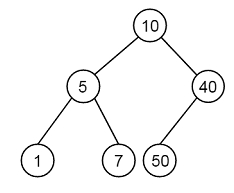# Construct BST from given preorder traversal - Set 1 in C++

Suppose we have one pre order traversal. From this traversal. we have to generate the tree So if the traversal is like [10, 5, 1, 7, 40, 50], then the tree will be like −To solve this, we will use this trick. The trick is to set a range {min… max} for each node. At first we will initialize the range as {INT_MIN… INT_MAX}. The first node will definitely be in range, so after that we will create root node. To construct the left subtree, set the range as {INT_MIN… root->data}. If a values is in the range {INT_MIN… root->data}, then the value is part of left subtree. To construct the right subtree, set the range as {root->data… max… INT_MAX}.

## Example

#include <iostream>
using namespace std;
class node {
public:
int data;
node *left;
node *right;
};
node* getNode (int data) {
node* temp = new node();
temp->data = data;
temp->left = temp->right = NULL;
return temp;
}
node* makeTreeUtil( int pre[], int* preord_index, int key, int min, int max, int size ) {
if( *preord_index >= size )
return NULL;
node* root = NULL;
if( key > min && key < max ){
root = getNode( key );
*preord_index += 1;
if (*preord_index < size){
root->left = makeTreeUtil( pre, preord_index, pre[*preord_index], min, key, size );
root->right = makeTreeUtil( pre, preord_index, pre[*preord_index],key, max, size );
}
}
return root;
}
node *makeTree (int pre[], int size) {
int preord_index = 0;
return makeTreeUtil( pre, &preord_index, pre, INT_MIN, INT_MAX, size );
}
void inord (node* node) {
if (node == NULL)
return;
inord(node->left);
cout << node->data << " ";
inord(node->right);
}
int main () {
int pre[] = {10, 5, 1, 7, 40, 50};
int size = sizeof( pre ) / sizeof( pre );
node *root = makeTree(pre, size);
cout << "Inorder traversal: ";
inord(root);
}

### Output

Inorder traversal: 1 5 7 10 40 50